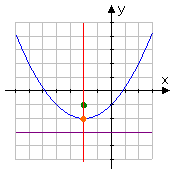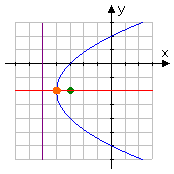Search

Conics: Parabolas: Introduction (page 1 of 4)

Sections: Introduction, Finding information from the equation, Finding the equation from information, Word problems & CalculatorsIn algebra, dealing with parabolas usually means graphing quadratics or finding the max/min points (that is, the vertices) of parabolas for quadratic word problems. In the context of conics, however, there are some additional considerations.

To form a parabola according to ancient Greek definitions, you would start with a line and a point off to one side. The line is called the "directrix"; the point is called the "focus". The parabola is the curve formed from all the points (x, y) that are equidistant from the directrix and the focus. The line perpendicular to the directrix and passing through the focus (that is, the line that splits the parabola up the middle) is called the "axis of symmetry". The point on this axis which is exactly midway between the focus and the directrix is the "vertex"; the vertex is the point where the parabola changes direction."regular", or vertical, parabola (in blue), with the focus (in green) "inside" the parabola, the directrix (in purple) below the graph, the axis of symmetry (in red) passing through the focus and perpendicular to the directrix, and the vertex (in orange) on the graph "sideways", or horizontal, parabola (in blue), with the focus (in green) "inside" the parabola, the directrix (in purple) to the left of the graph, the axis of symmetry (in red) passing through the focus and perpendicular to the directrix, and the vertex (in orange) on the graph

The name "parabola" is derived from a New Latin term that means something similar to "compare" or "balance", and refers to the fact that the distance from the parabola to the focus is always equal to (that is, is always in balance with) the distance from the parabola to the directrix. In practical terms, you'll probably only need to know that the vertex is exactly midway between the directrix and the focus.

In previous contexts, your parabolas have either been "right side up" or "upside down" graph, depending on whether the leading coefficient was positive or negative, respectively. In the context of conics, however, you will also be working with "sideways" parabolas, parabolas whose axes of symmetry parallel the x-axis and which open to the right or to the left.

A basic property of parabolas "in real life" is that any light or sound ray entering the parabola parallel to the axis of symmetry and hitting the inner surface of the parabolic "bowl" will be reflected back to the focus. "Parabolic dishes", such as "bionic ears" and radio telescopes, take advantage of this property to concentrate a signal onto a receiver. The focus of a parabola is always inside the parabola; the vertex is always on the parabola; the directrix is always outside the parabola.

The "general" form of a parabola's equation is the one you're used to, y = ax2 + bx + c  unless the quadratic is "sideways", in which case the equation will look something like x = ay2 + by + c. The important difference in the two equations is in which variable is squared: for regular (vertical) parabolas, the x part is squared; for sideways (horizontal) parabolas, the y part is squared.

The "vertex" form of a parabola with its vertex at (h, k) is:

regular: y = a(x  h)2 + k

The conics form of the parabola equation (the one you'll find in advanced or older texts) is:

regular: 4p(y  k) = (x  h)2
sideways: 4p(x  h) = (y  k)2

Why "(h, k)" for the vertex? Why "p" instead of "a" in the old-time conics formula? Dunno. The important thing to notice, though, is that the h always stays with the x, that the k always stays with the y, and that the p is always on the unsquared variable part. The relationship between the "vertex" form of the equation and the "conics" form of the equation is nothing more than a rearrangement:

y = a(x  h)2 + k
y  k = a(x  h)2
(1/a)(y  k) = (x  h)2
4p(y  k) = (x  h)2

In other words, the value of 4p is actually the same as the value of 1/a; they're just two ways of saying the exact same thing. But this new variable p is one you'll need to be able to work with when you're doing parabolas in the context of conics: it represents the distance between the vertex and the focus, and also the same (that is, equal) distance between the vertex and the directrix. And 2p is then clearly the distance between the focus and the directrix.

Regardless of whether a given parabola is the "reqular" type or a sideways one, you can always find the intercepts in the usual way; namely, for the x-intercept(s) (if any), plug 0 in for y and solve, and for the y-intercept(s) (if any), plug 0 in for x and solve.

• State the vertex and focus of the parabola having the equation (y  3)2 = 8(x  5).
• Comparing this equation with the conics form, and remembering that the h always goes with the x and the k always goes with the y, I can see that the center is at (h, k) = (5, 3). The coefficient of the unsquared part is 4p; in this case, that gives me 4p = 8, so p = 2. Since the y part is squared and p is positive, then this is a sideways parabola that opens to the right. The focus is inside the parabola, so it has to be two units to the right of the vertex:

vertex: (5, 3); focus: (7 3)

• State the vertex, the directrix, and any intercepts of the parabola having the equation (x + 3)2 = 20(y  1).
• The temptation is to say that the vertex is at (3, 1), but that would be wrong. The conics form of the equation has subtraction inside the parentheses, so the (x + 3)2 is really (x  (3))2, and the vertex is at (3, 1).

The coefficient of the unsquared part is 20, and this is also the value of 4p, so p = 5. Since the x part is squared, this is a "regular" parabola; and since the value of p is negative, then this parabola opens downward. Since the directrix is on the outside of its parabola, and since this parabola is vertical and opening downward, then the directrix must be five units above the vertex. Since the y-value of the vertex is 1, then the directrix is at 1 + 5 = 6.

To find the intercepts, we plug in zeroes in for our variables. (Note: While a given "regular" parabola will always eventually cross the y-axis, it might not cross the x-axis. For instance, if the vertex of this parabola had been below the x-axis, then the curve would never have crossed, and there would be no x-intercepts.)

For the x-intercepts, we plug zero in for y, which, after some rearranging, gives us the quadratic equation x2 + 6x - 11 = 0. Applying the Quadratic Formula, we get the following x-intercepts:

...or about x = −7.5 and x = +1.5. For the y-intercept, we plug zero in for x and solve, which gives us y = 11/20 = 0.55.

vertex: (3, 1)
directrix: y = 6
intercepts: (-7.5, 0), (+1.5, 0), (0, 0.55)

Top  |  1 | 2 | 3 | 4  |  Return to Index  Next >>

 Cite this article as: Stapel, Elizabeth. "Conics: Parabolas: Introduction." Purplemath. Available from     https://www.purplemath.com/modules/parabola.htm. Accessed [Date] [Month] 2016

Study Skills Survey

Tutoring from Purplemath
Find a local math tutor